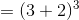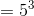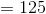# PSAT Math : How to find a rational number from an exponent

## Example Questions

### Example Question #232 : Exponents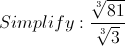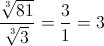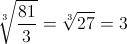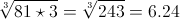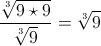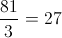Explanation: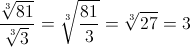### Example Question #1 : How To Find A Rational Number From An Exponent

Rationalize the denominator: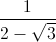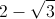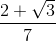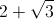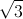Explanation:

The conjugate ofis.

Now multiply both the numerator and the denominator byand you get: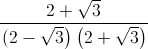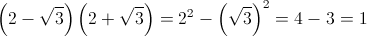Hence we get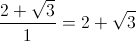### Example Question #234 : Exponents

Solve for: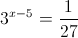Explanation: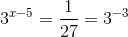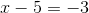### Example Question #235 : Exponents

Solve for.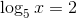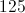Explanation: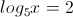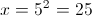### Example Question #1 : How To Find A Rational Number From An Exponent

If,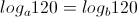What does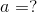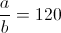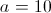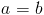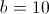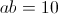Explanation:

If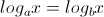,

then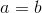.

### Example Question #237 : Exponents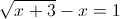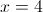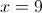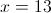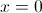Explanation:

From the equation in the problem statement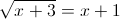Now squaring both sides we get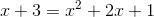this is a quadratic equation which equals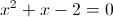and the factors of this equation are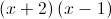This gives us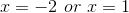.

However, if we plug these solutions back into the original equation,does not create an equality. Therefore,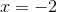is an extraneous solution.

### Example Question #1 : How To Find A Rational Number From An Exponent

For some positive integer, if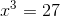, what is the value of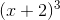?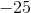Explanation:

If, thenmust equal 3 (Note thatcannot be -3 because you need it to be positive.

Now, pluginto the new equation: